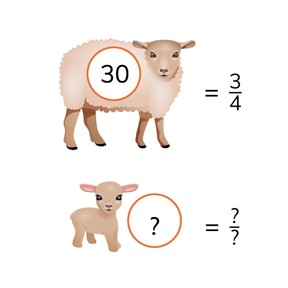Determine the missing portion of a set using a fraction

# Determine the missing portion of a set using a fraction

I can determine the missing portion of a set using a fraction.

No account needed.8,000 schools use Gynzy92,000 teachers use Gynzy1,600,000 students use Gynzy

## General

Students learn to determine the missing amount of a portion by using a fraction.

## Common core standard(s)

CCSS.MATH.CONTENT.4.NF.A.1
CCSS.MATH.CONTENT.5.NF.B.4

## Relevance

It is important to learn how to do this, so you are able to calculate missing portions and then can express that amount as a fraction.

## Introduction

Students are asked to add fractions to equal 1 or to subtract from 1 with fractions.

## Development

Refresh with students that fractions are made of a numerator and denominator. Show the problem and tell students that 1//3 of the whole class is made up of 7 boys. Explain that you first look to determine which fraction is missing. 3//3 - 1//3 = 2//3.
You now know that 2//3 of the class is girls. You also know that 1//3 equals 7. You multiply the numerator with the amount of the missing portion. 7 x 2 = 14. You now know that there are 14 girls in class and 7 boys. Emphasize the fact that you have to find two answers here, first 2//3 and then 14. Go through the same steps with the problem with frogs. Check with the following problem that students are able to determine the missing portion of the set by using a fraction. Next, explain to students how to solve a fraction word problem. Make sure that you first determine which numbers you need from the story and then to say or write the problem before solving. Use the next fraction story problem to check that students are able to determine the missing portion of a set by using a fraction.

Check that students are able to determine the missing portion of a set using a fraction by asking the following question:
- Which steps do you take to determine the missing part of a set with a fraction?

## Guided practice

Students are given multiple choice questions along with a fraction bar to help them determine the answer. They then must fill in the answer when given a fraction bar. Finally they are given story problems in which they must determine the total.

## Closing

Discuss with students the importance of being able to determine the missing portion of a set by using a fraction. To close ask students to create their own math problem that has a fraction in small groups. When they have created their problem, another group must come to solve the problem. You can use the prompt from the interactive whiteboard to help students with creating a math problem. For example: In 1//3 part of the gym there are 12 boys. How many girls are in the gym and what fraction is that?

## Teaching tips

Students who have difficulty with determining the missing part of a set using a fraction can first practice determining fractions. For example: How many more must you add to 1//4 to make a whole? You can use fraction circles or bars as a visual/manipulative.

### The online teaching platform for interactive whiteboards and displays in schools

• Save time building lessons

• Manage the classroom more efficiently

• Increase student engagement